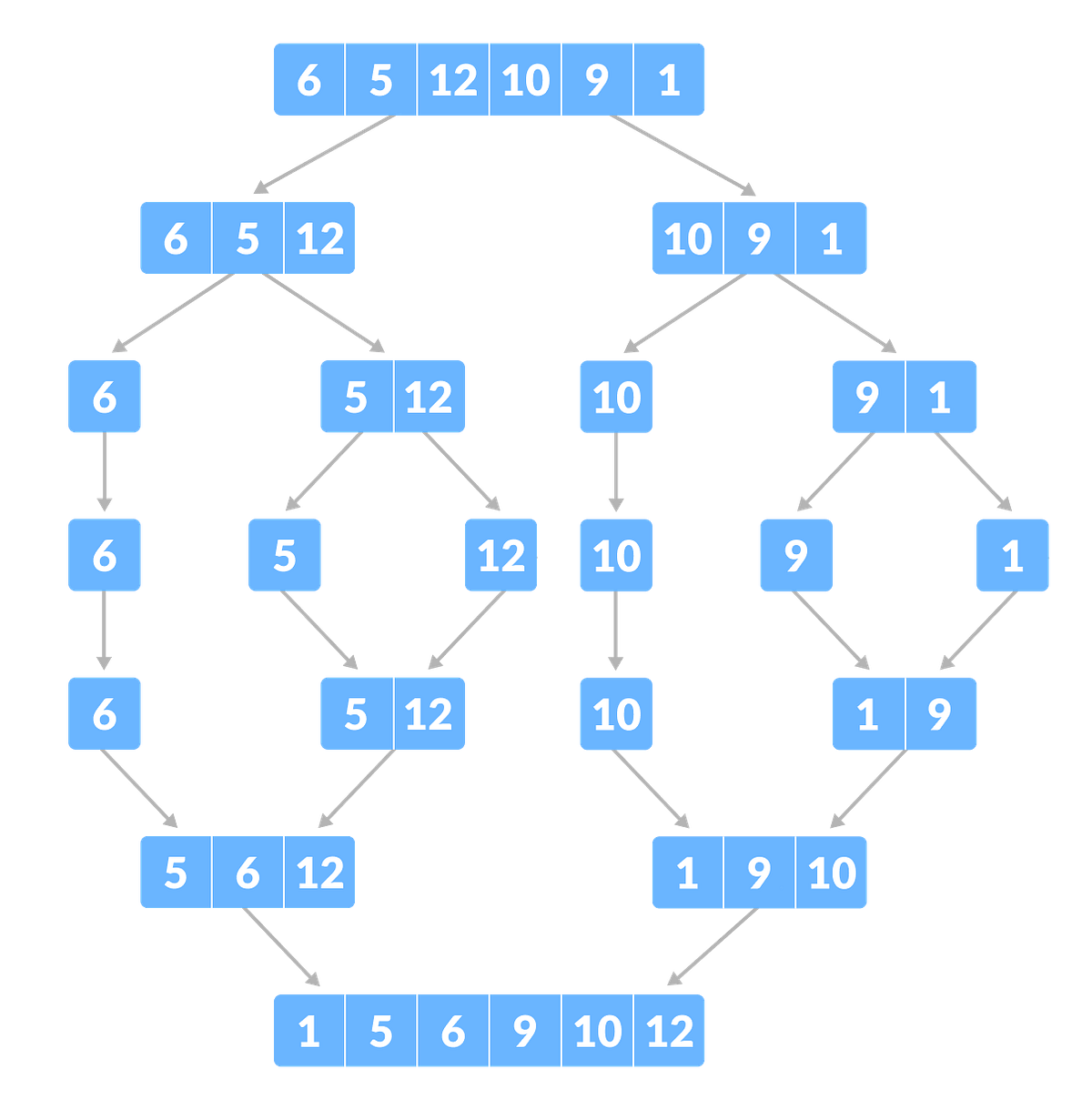# Merge Sort Algorithm in JavascriptIn this post we will learn Merged Sort Algorithm in Javascript . Sorting is rearranging a set of data into a specific order such as ascending to descending for numbers, or vice versa. A sorting algorithm takes in a given array or list and outputs a sorted array or list.

Sorting is rearranging a set of data into a specific order such as ascending to descending for numbers, or vice versa. A sorting algorithm takes in a given array or list and outputs a sorted array or list.

Sorting is applied everywhere. When we are on a website and sorting listings by lowest price to highest, ratings, etc. or searching for a book at the library. The concept of sorting makes it easier to arrange sets of data, therefore making it easier to search.

## Types of Sorting Algorithms

There are many types of sorting algorithms. Sorting techniques are mainly differentiated by time complexity and space complexity. As the name implies, time complexity is the amount of time an algorithm takes to run as a function of the input length and space complexity is the amount of space or memory required as a function of input length. Below lists a a few different types of sorting algorithms:

• Quick sort
• Bubble sort
• Merge sort
• Insertion sort
• Heap sort

## How to do Problem Solving as a Developer

In this video, I will be talking about problem-solving as a developer 🔴 Subscribe for more https://www.youtube.com/channel/UCMA8gVyu_IkVIixXd2p18NQ?sub_confi...

## What Kind of Problems Can Quantum Computers Solve?

And What Kind of Problems Can’t They Solve? Before we get into the good stuff, I need to tell you that quantum computers are not magical.

## Problem Solving Patterns to know JavaScript

These patterns won’t help you solve every problem you’ll face, but they can improve your chances significantly. This article will cover the first three: frequency counter, multiple pointers, and sliding window. First, let’s talk about…

## While You Don't Understand Recursion, Read Recursion: by Randy Taylor

Recursion is the one idea I constantly use while I solve coding problems. Most of the time I don’t start by thinking “RECURSION WILL SOLVE THIS!”. However recursion just ends up being the logical way to reach an answer.

## Algorithm Problem Solving — Recursion Basics

Algorithm Problem Solving — Recursion Basics. We are going to talk about how to intuitively think about recursion.